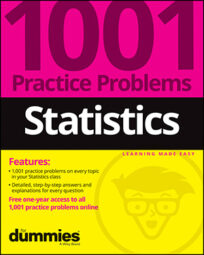##### Statistics: 1001 Practice Problems For Dummies (+ Free Online Practice)When you look at the difference between two means (or two proportions), keep track of what populations you're calling Population 1 and Population 2. Subtracting two numbers in the opposite order changes the sign of the results!

A random sample of 120 college students who were physics majors found that they spent an average of 25 hours a week on homework; the standard deviation for the population of physics majors was 7 hours. A random sample of 130 college students who were English majors found that they spent an average of 18 hours a week on homework; the standard deviation for the population of English majors was 4 hours.

Call the population of physics majors "Population 1" and the population of English majors "Population 2" while working these problems.

## Sample questions

1. If you did not know the population standard deviations, how would your calculation of confidence intervals differ?

(A) You would use t* from a t-distribution rather than z* from the standard normal distribution.

(B) You would use the sample standard deviations rather than the population standard deviations.

(C) You would combine the sample sizes and divide the sum of the standard deviations by (n1 + n2).

(D) Choices (A) and (B)

(E) Choices (A), (B) and (C)

Answer: D. Choices (A) and (B) (You would use t* from a t-distribution rather than z* from the standard normal distribution; you would use the sample standard deviations rather than the population standard deviations.)

If you're estimating the difference in two population means and don't know the population standard deviations, you use t* rather than z* and the sample standard deviations when calculating a confidence interval.

2. If your sample sizes were 35 English majors and 20 physics majors, how, if at all, would your calculation of confidence intervals differ?

Answer: You would use a t*-value rather than a z*-value.

If you're estimating the difference in two population means and one or both of your sample sizes are less than 30, you use a t*-value from a t-distribution rather than a z*-value from a standard normal distribution when calculating a confidence interval.

If you need more practice on this and other topics from your statistics course, visit 1,001 Statistics Practice Problems For Dummies to purchase online access to 1,001 statistics practice problems! We can help you track your performance, see where you need to study, and create customized problem sets to master your stats skills.